# Electron Quantum NumbersPage 2

#### WATCH ALL SLIDES

Shape of f (l = 3) orbitals

Slide 11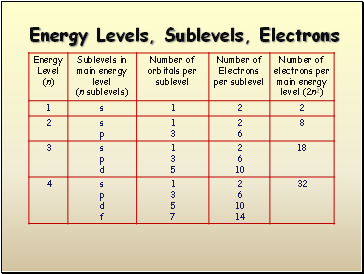## Energy Levels, Sublevels, Electrons

Slide 12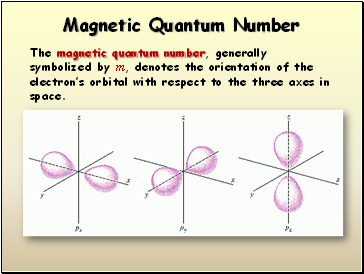## Magnetic Quantum Number

The magnetic quantum number, generally symbolized by m, denotes the orientation of the electron’s orbital with respect to the three axes in space.

Slide 13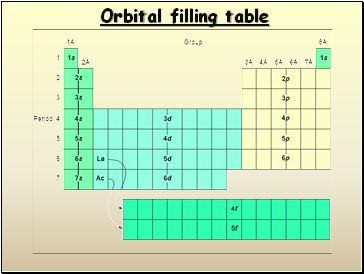## Orbital filling table

Slide 14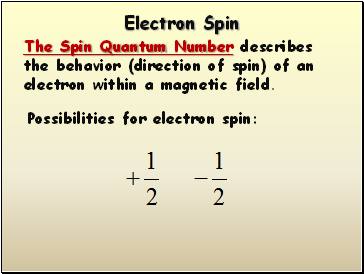## Electron Spin

The Spin Quantum Number describes the behavior (direction of spin) of an electron within a magnetic field.

Possibilities for electron spin:

Slide 15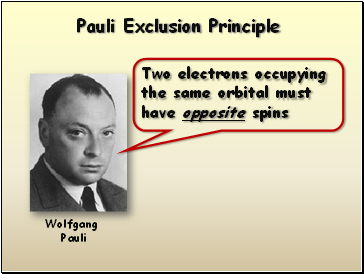## Pauli Exclusion Principle

Two electrons occupying the same orbital must have opposite spins

Wolfgang

Pauli

Slide 16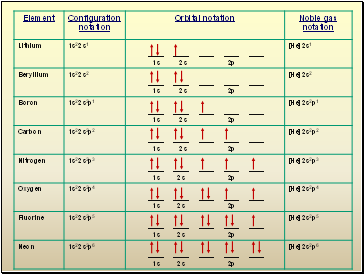Slide 17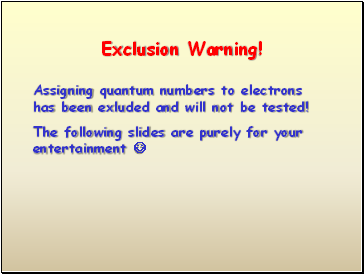## Exclusion Warning!

Assigning quantum numbers to electrons has been exluded and will not be tested!

The following slides are purely for your entertainment 

Slide 18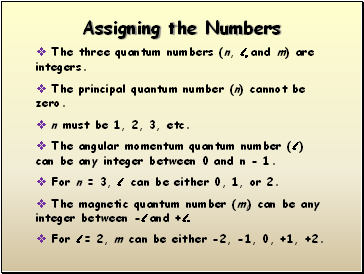## Assigning the Numbers

The three quantum numbers (n, l, and m) are integers.

The principal quantum number (n) cannot be zero.

n must be 1, 2, 3, etc.

The angular momentum quantum number (l ) can be any integer between 0 and n - 1.

For n = 3, l can be either 0, 1, or 2.

The magnetic quantum number (ml) can be any integer between -l and +l.

For l = 2, m can be either -2, -1, 0, +1, +2.

Slide 19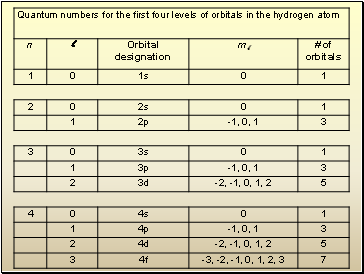Go to page:
1  2